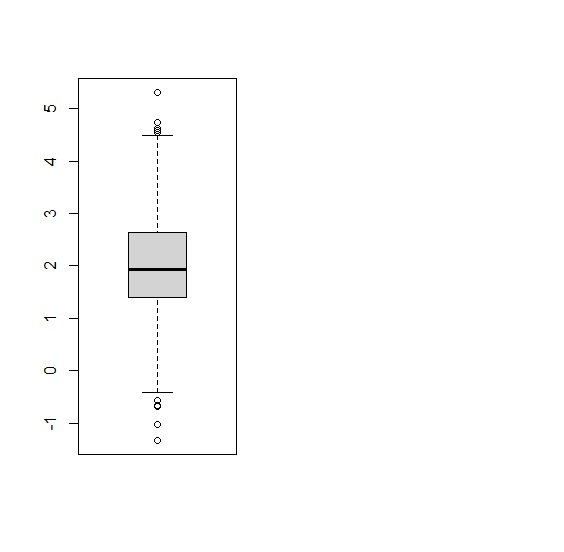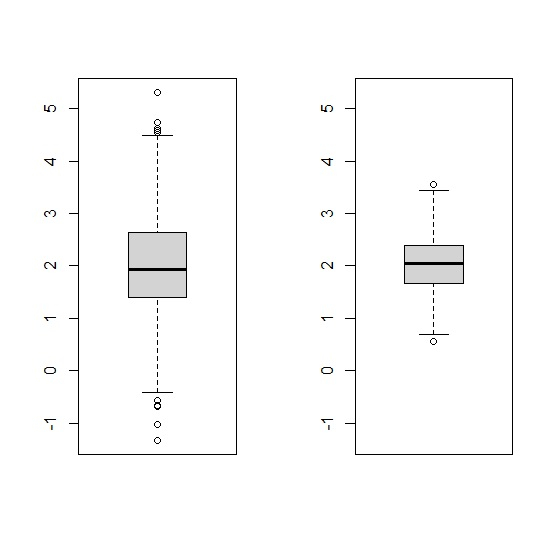# How to create side-by-side boxplot in base R?

Often, we need to compare continuous variables using boxplots and thus side-by-side boxplots are required. Creating side-by-side boxplot in base R can be done with the help of creating space for graphs with the help of par(mfrow=). In this function, we can define the number of graphs and the sequence of these graphs, thus creation of side-by-side boxplot will become easy.

Consider the below vectors −

set.seed(100)
x<-rnorm(500,2,1)
y<-rnorm(500,2,0.5)

Define the range for Y-axis −

Y_range<-range(x,y)

Creating graph space using par(mfrow=) −

par(mfrow=c(1,2))

Creating first boxplot −

boxplot(x,ylim=Y_range)


This will create below output −Creating second boxplot −

boxplot(y,ylim=Y_range)